Vibroengineering PROCEDIA

Published: 15 March 2019

# Numerical investigation for influence of pre-twist on stress behavioral characteristics of curved blade

Chandra Sekhar Akula2
Himam Saheb Shaik3
1, 2, 3Department of Mechanical Engineering, Faculty of Science and Technology, IFHE, Hyderabad, India
Corresponding Author:
Views 104

#### Abstract

To assist designers in developing blade design based on conventional and composite materials, an overview of material characteristics and stress analysis methods was taken for consideration. The significant effect of loading on the pre-twisted curved blade are evaluated in terms of its deflection and corresponding stresses. A more simplified approach was not satisfactory and furthermore to establish the feasibility of composite blade detailed stress analysis has been carried out for various pre-twist angles of composite and mild steel blade using a thick shell element. The suitability of the finite element method in predicting the stresses in externally loaded laminates is examined from the viewpoint of satisfaction of all boundary conditions and results. From the results it can be shown that the three-dimensional finite element analysis provides a useful insight for predicting deflection and stresses. The influence of ply angle for the distribution of inter laminar stresses along $y$ and $z$-axis are determined.

## 2. Material and methods

The main objective of the present research work emphasizes on the study of stress behavioral characteristics of the curved blade tested with both conventional and composite materials. Application of composite materials are more suitable than isotropic materials, which is mainly due to the high strength to weight ratio. The following Tables 1 and 2 gives the cross-section details and material properties of conventional and composite material used for curved blade analysis.

Table 1Material properties and dimensions of Titanium blade

 Blade dimensions Material BC and loading 3 and 5 mm thick (at sides) Titanium Ti-6Al-4 Fixed: left face 8 mm thick (at the middle) Modulus = 113.8 GPa 50 mm wide (base and top) Poisson’s ratio = 0.342 150 mm long Yield stress = 950 MPa 18° tilt angle (base s tip) Density = 4430 kg/m3

Table 2Material properties of composite for curved blade

 Material ${E}_{x}$(GPa) ${E}_{y}$(GPa) ${E}_{z}$(GPa) ${\upsilon }_{xy}$ ${\upsilon }_{yz}$ ${\upsilon }_{zx}$ ${G}_{xy}$(GPa) ${G}_{yz}$(GPa) ${G}_{zx}$(GPa) Density (g/cc) S-glass fabric/epoxy 22.925 22.925 12.4 0.12 0.2 0.2 4.7 4.2 4.2 1.8

## 3. Results and discussions

In this present study the effect of pre-twist, fiber orientations and layer lamination on the stress analysis of a twisted blade are considered. In order to find out the stress behaviour response for the blade, shell 181 elements are used for finite element modelling and the results are varied in steps of twist angles from 0 to 90°. The corresponding tables shows the quantative comparisons of the various stresses and deflections of a non- rotating blade. The blade is meshed up with shell elements using Hypermesh solver and the numerical results are obtained using ANSYS. The Table 3, 4 shows the Stress values of Titanium and composite curved blade for various pre-twist angles and the corresponding graphs 2a, 2b represents the stress values as a function of pre-twist angles. The Table 5 shows the variation in stresses of a pre-twisted composite blade drawn with varying number of layers at an angle of 18°. Finally, Table 6 shows the results for inter laminar stresses of composite blades with various pre-twist angles and number of layers.

Fig. 1Pre-twisted single blade with pressure distribution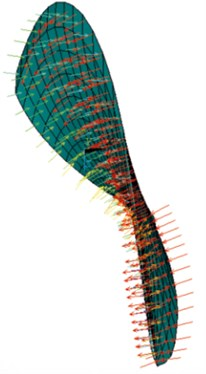Table 3Stress values of titanium curved blade for various pre-twist angles

 Parameter $\alpha =$ 0 $\alpha =$ 15 $\alpha =$ 18 $\alpha =$ 30 $\alpha =$ 45 $\alpha =$ 60 Deflection (mm) 0.431e-3 0.431e-3 0.431e-3 0.432e-3 0.432e-3 0.432e-3 $X$-component (MPa) 275.324 270.331 277.377 265.321 260.265 255.351 $Y$-component (MPa) 216.179 219.184 209.907 213.407 208.902 206.149 $Z$-component (MPa) 308.291 295.294 293.015 285.192 278.984 277.757 I-Principal (MPa) 32.575 15.626 11.969 13.14 21.84 38.328 II-Principal (MPa) 213.407 208.455 207.58 204.463 201.541 199.947 III-Principal (MPa) 563.418 560.727 560.751 561.944 564.853 570.269 Von Mises (MPa) 658.220 657.495 656.969 653.652 647.108 638.656

Table 4Stress values of the composite curved blade for various pre-twist angles

 Parameter $\alpha =$ 0 $\alpha =$ 15 $\alpha =$ 18 $\alpha =$ 30 $\alpha =$ 45 $\alpha =$ 60 Deflection (mm) 0.007331 0.007331 0.007331 0.007333 0.007337 0.00734 $X$-component (MPa) 231.568 231.568 237.791 227.234 223.852 220.353 $Y$-component (MPa) 204.666 204.666 198.082 203.847 201.762 199.368 $Z$-component (MPa) 365.034 365.034 342.402 356.148 350.502 349.275 I-Principal (MPa) 28.996 28.996 26.078 13.671 20.147 16.492 II-Principal (MPa) 201.200 201.2 199.67 198.333 196.335 194.889 III-Principal (MPa) 575.043 575.043 574.975 576.818 581.928 590.599

Table 5Stress values of the composite curved blade for varying number of layers

 Parameter 4 layers 8 layers 16 layers 24 layers Deflection (mm) 0.007326 0.007328 0.007330 0.007331 $X$-component (MPa) 256.421 231.568 230.099 228.631 $Y$-component (MPa) 221.498 204.666 200.579 196.492 $Z$-component (MPa) 413.084 365.034 366.744 368.454 I-Principal (MPa) 98.968 28.996 27.656 26.316 II-Principal (MPa) 223.580 201.200 200.413 199.627 III-Principal (MPa) 580.137 575.043 578.008 580.973

Table 6Inter laminar stress values of composite curved blade for various number of layers

 No. of layers ILSXZ (MPa) ILSYZ (MPa) 4 122.691 221.148 8 113.192 249.109 16 111.111 252.073 24 109.031 255.037

Fig. 2Stress values comparison for various pre-twist angles for isotropic and composite material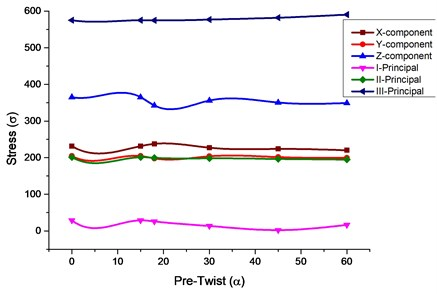a) Isotropic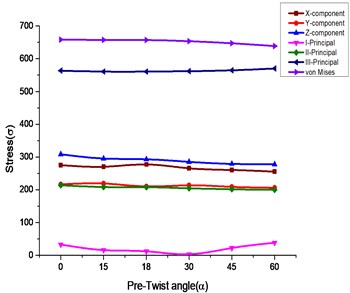b) Composite

Fig. 4Stress value comparison for varying layers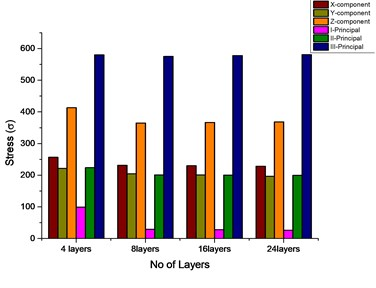Fig. 5Inter laminar stress comparison for various pre-twist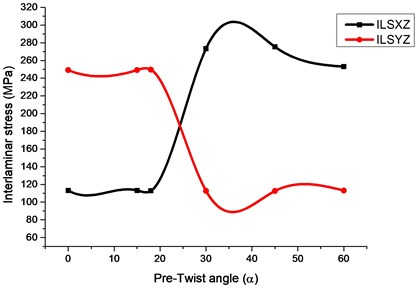## 4. Conclusions

In this study the solid model of the curved blade is developed using CATIA, the finite element modelling is carried out using Hyper mesh and the analysis results are interpreted by using FEA based simulation software ANSYS 15, subjected under clamped-free conditions. The blade was modelled as a cantilever oriented arbitrarily with respect to the rotating axes. The effects of initial twist, fiber orientation, and composite laminates were investigated for finding out the deflection, Principal stresses, von Misses stress XYZ component stress and corresponding inter laminar stresses are determined and corresponding graphs are plotted. Finally, a comparison has been made between isotropic and composite. From the results, it can be predicted by proper tailoring composite materials at specified ply angles and varying the number of layers stresses and deflections can be enhanced to cope up with isotropic materials.

1. From Table 3 it shows that the XYZ component of stress for an isotropic titanium curved blade lies maximum for a pre-twist angle between 0 to 30°.The principal component of stresses first and third principal stress are maximum at pre-twist angle of 60°.The second principal stress and von Mises stress are maximum for pre-twist angle of 0°.

2. From Table 4 the XYZ and principal component of stress (First and Second) for a composite curved blade lies maximum for a pre-twist angle of 0°. The third principal component of stress is maximum at pre-twist angle of 60°.

3. From Table 5, 6 it shows that with increasing number of layers from 4 to 24 a reduction in stress values are obtained for both principal component and inter laminar stresses and are depicted in Figure 4. With varying pre-twist angles from 0 to 60° in Figure 5 an incremental growth of inter-laminar stress along XZ axis and reduction along YZ axis can be observed.

#### Cited by

Study on Design and Analysis of Industrial Y Type Strainer